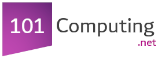Start

End
Create a program that asks the user to enter three whole numbers.
The program will display the average of these three numbers.
num1 = int(input("Type a number:"))
num2 = int(input("Type another number:"))
num3 = int(input("Type a third number:"))
average = (num1 + num2 + num3) / 3
print("The average is " + str(average))